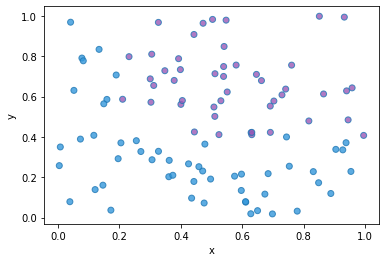# Control Color of Each Marker

This post displays an example scatterplot built with seaborn to show how to control each marker's color by creating a new column in the dataset.

The previous posts control marker features and map a categorical value to a color show how to control the color of all markers or the markers of specific categories in the data. However, it is also possible to control each marker's color in the plot. You will see how to have a more precise control on the color in this example. Dataset is created with random points. In order to control colors, a new column is build with the desired color for each marker (data point).

``````# libraries
import pandas as pd
import numpy as np
import matplotlib.pylab as plt
import seaborn as sns

# Create data frame with randomly selected x and y positions
df = pd.DataFrame(np.random.random((100,2)), columns=["x","y"])

# Add a column: the color depends on x and y values, but you can use any function you want
value=(df['x']>0.2) & (df['y']>0.4)
df['color']= np.where( value==True , "#9b59b6", "#3498db")

# plot
sns.regplot(data=df, x="x", y="y", fit_reg=False, scatter_kws={'facecolors':df['color']})

plt.show()``````## Contact & Edit

👋 This document is a work by Yan Holtz. Any feedback is highly encouraged. You can fill an issue on Github, drop me a message onTwitter, or send an email pasting `yan.holtz.data` with `gmail.com`.